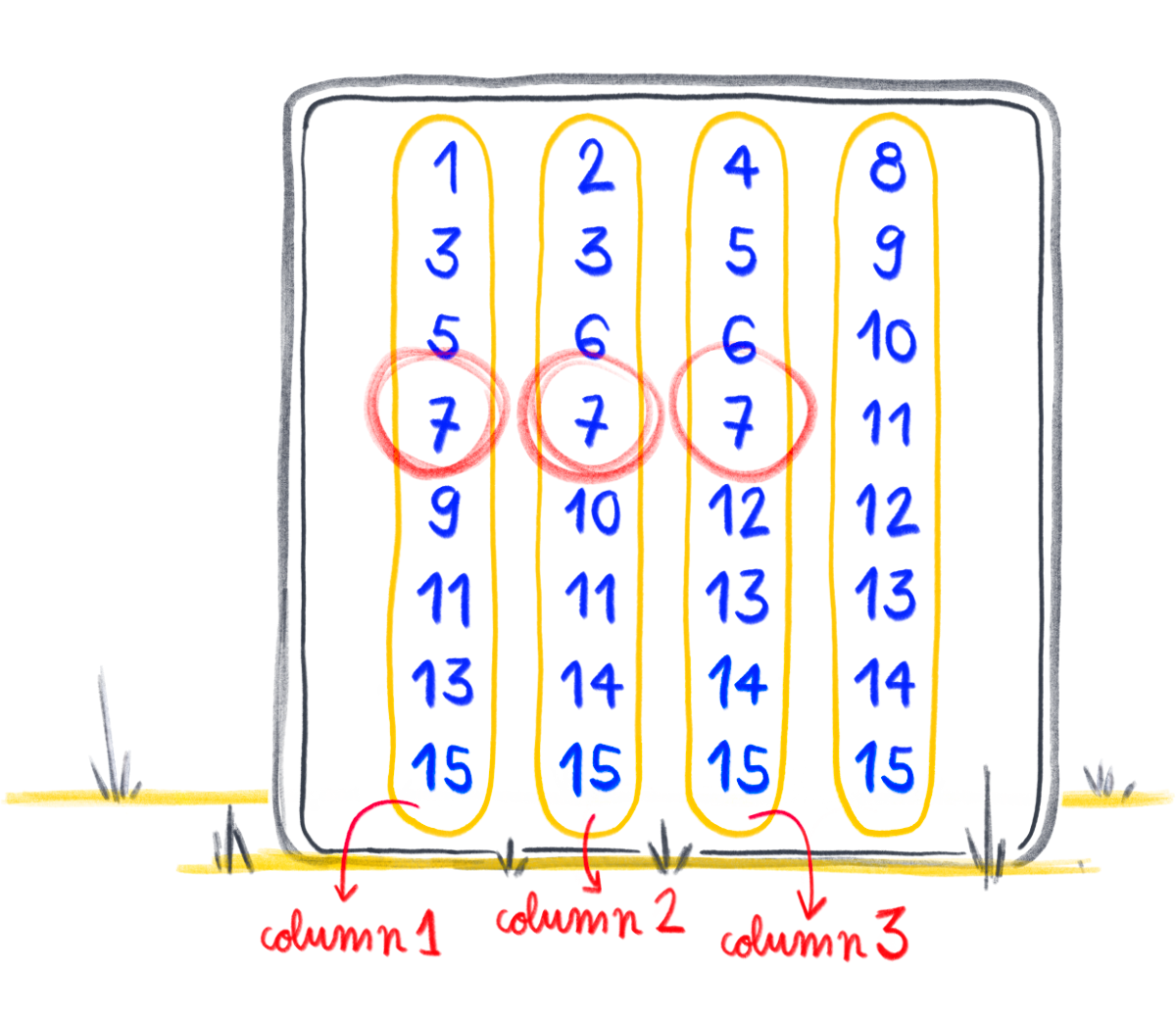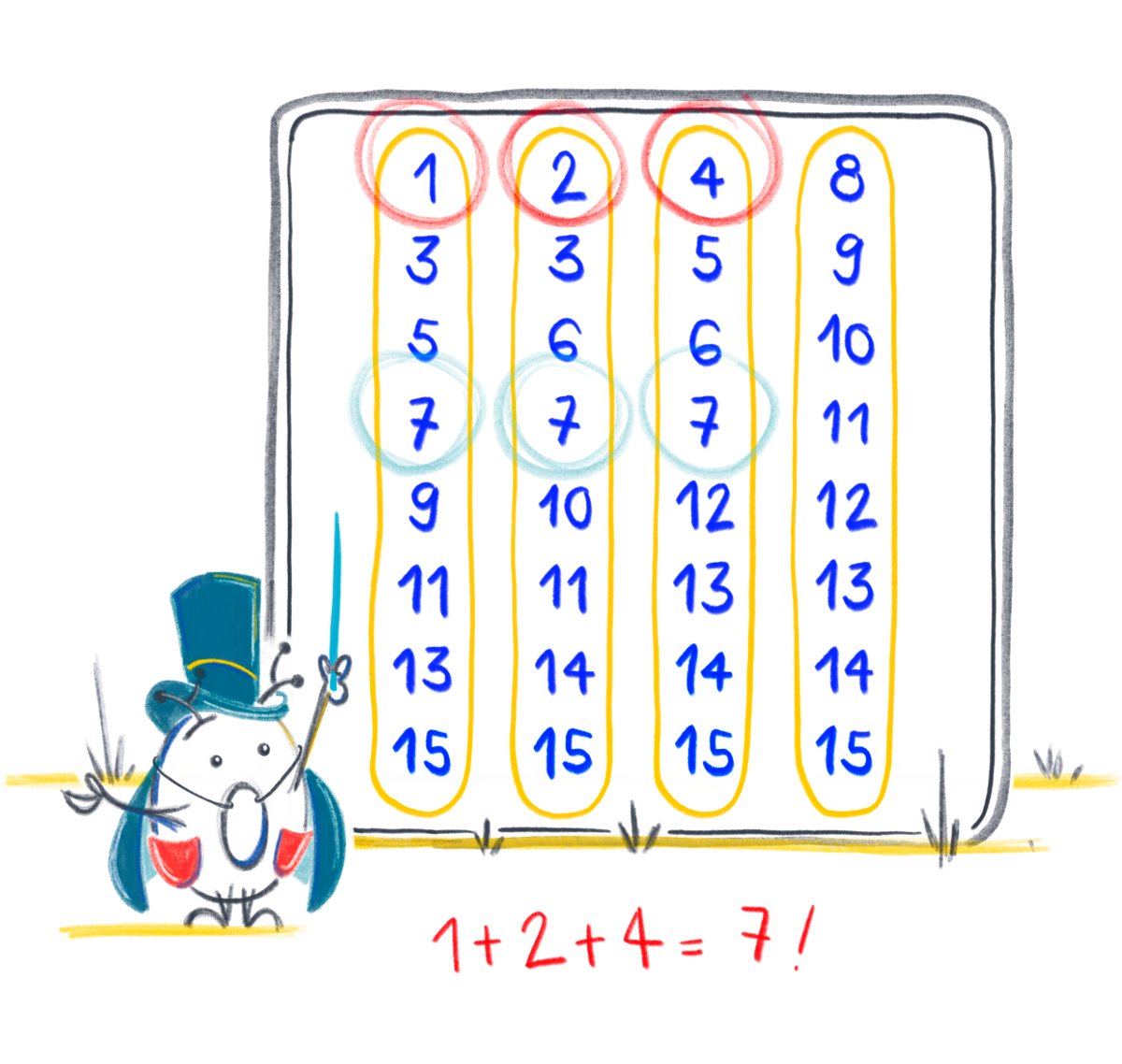# Binary Magic

by Alicia TocinoMathematics and magic are often seen as independent disciplines but actually, they play together! How can we learn about binary numbers with a touch of magic?

## Preparation

Get ready for your children and write the following 4 columns of numbers on your whiteboard or on a blank piece of paper.## Instructions

Start by asking your children to think of a number between 1 and 15 and to keep it for themselves.One by one, ask each of them to tell you the columns in which their numbers appear.Now sum up the first numbers in each of the columns. Is that the number they had in their minds?The result you've obtained matches the number that your children had thought of and they'll be amazed! 💫

Important: You need to guess the number without looking at the columns of numbers. Otherwise, your little Humans will think you're cheating!

## But, is this magic?No, it's mathematics!

We can decompose any number as a sum of powers of 10. Let's remember that the powers of 10 are of the form:

100 = 1

101 = 10

102 = 100

103 = 1000

...

That means that we can decompose the number 247 as 200 + 40 + 7. In turn, we can factor each of the previous addends:

200 = 2 x 100 = 2 x 102
40 = 4 x 10 = 4 x 101
7 = 7 x 1 = 7 x 100

Finally we have:

247 = 2 x 102 + 4 x 101 + 7 x 100

What happens if we use now powers of 2? Let's remember what the powers of two are:

20 = 1

21 = 2

22 = 4

23 = 8

...

We can express any number as the sum of these powers and thus obtain its representation as a binary number.

Let's look at some examples:

1 = 0 x 23 + 0 x 22 + 0 x 21 + 1 x 20 => 0001

2 = 0 x 23 + 0 x 22 + 1 x 21 + 0 x 20 => 0010

3 = 0 x 23 + 0 x 22 + 1 x 21 + 1 x 20 => 0011

4 = 0 x 23 + 1 x 22 + 0 x 21 + 0 x 20 => 0100

5 = 0 x 23 + 1 x 22 + 0 x 21 + 1 x 20 => 0101

11 = 1 x 23 + 0 x 22 + 1 x 21 + 1 x 20 => 1011

As it happens in the decomposition into powers of 10, we order the powers from the highest to the lowest exponent.

### What does all this have to do with the trick I just learned?

I'm glad you asked! The key is in the way that we organize the numbers in the columns. The numbers at the top of each column correspond to:

20 = 1

21 = 2

22 = 4

23 = 8

A number goes in the column of 20 if in its decomposition as sums of powers of two a 1 appears multiplying by 20.

It should be included in the column headed by 21 if a 1 appears multiplying by 21 in the decomposition. And so on until all the columns are completed.

For this reason, the number 7, which is decomposed like:

7 = 0 x 23 + 1 x 22 + 1 x 21 + 1 x 20

only appears in the 20, 21, and 22 columns.

## Extra Challenge

Would you be able to add one more column, headed by 24 = 16, and add the numbers 16 through 31 to keep the trick working?

##### Solution
Column 1: 1,3,5,7,9,11,13,15,17,19,21,23,25,27,29,31
Column 2: 2,3,6,7,10,11,14,15,18,19,22,23,26,27,30,31
Column 3: 4,5,6,7,12,13,14,15,20,21,22,23,28,29,30,31
Column 4: 8,9,10,11,12,13,14,15,24,25,26,27,28,29,30,31
Column 5: 16,17,18,19,20,21,22,23,24,25,26,27,28,29,30,31

## Notes

• Mathematics: Number – Exploring numbers in other bases. Representing numbers in base two.
• Mathematics: Algebra – Continue a sequential pattern, and describe a rule for this pattern. Patterns and relationships in powers of two.

#### Materials

• Whiteboard or a blank piece of paper
• Pen or pencil to write the columns of numbers